The outline (autoreferat) of my PhD, published in 1972.
in CORDIC field

Hardware implementation of the elementary functions  by digit-by-digit (CORDIC) technique

PhD Thesis,

On page 3   I say about optimal relationship between software and hardware
(codesign, as we say now). Then - about a problem of the algorithms choice
for hardware elementary functions evaluation.
On page 4: it is written about main characeristics of the hardware implemen-
tation: fast convergence, high accuracy, required time , and required
hardware. Two methodes will be considered here: digit by digit (mainly)
and polynomial approximation (for comparison).
Then I say about conversion , based on which digit by digit method is actually
founded:   from radix  a   and weights   a**(-i) to the weights:  ln(1+-a**(-i)
or to arctan (a**-i)  or to Arth(a**-i) where these values are the radices and
a=2,10...
On page 5 you will find three conditions of the convergence of the digit
by digit algorithms and the ways of sign operators choice: {0,1] or
{+1,-1}.
On page 6  the word "double " iterations (in Russian: "dvojnie"
iteratsii) is firstly appeared. I suggest them for ln  and  exp  functions.
On page 7 I suggest double iterations for convergence of arcsine and
arscosine functions and also for square root .
Then I propose to consider Volder's and Meggitt's algorithms as two modi-
fications of digit by digit method.( That is why I rarely use the word
"CORDIC").
On the lower passage of page 7   I  enumerate the main distinctions between
Volder and Meggitt algorithms.
On page 8 there is a table 1, generalized recurrence equations for both
approaches.
On page 9 it is noticed that in the thesis also considered digit by digit
method application for 2-10  1nd  10-2 conversions and for solving
systems of equations, analysed their convergence.
Then I say that all the second chapter is related to error analysis.
In outline a show it on the example of sine and cosine evaluation.
Pages 10, 11 and tables 2 and 3 related to description of error analysis.
On page 12 I describe how to analyze errors of multiplication operation
and errors of polynomial evaluation, based on Horner's rule.
Integral on page 14 I use for estimate of the mean value of the polynomial
evaluation error.
On page 15 I suggest how to choose optimal polynomial power, based on
relationship between polynomial approximation and aruthmetic rounding
errors.
Then I compare hardware implementation of digit by digit and polynomial
methods.
On page 16 I notice that derivative of the time curve , required for
doigit by digit method decreases, when word size increases. In contrast
with that for polynomial approximation the derivative is approximately
constant. It means that relative efficient of digit by digit method
increases with the word size.
Then I describe the results of an experimental statistical analysis of
digit by digit method accuracy.
On page 17 I describe the details of that analysis: I vary number
of iterations, word size, sample size. Results - histogramms, determi-
nation of kind of distribution law, and also root mean square
of errors for different values of word size.
In the end of page 17 and on the page 18 I describe the results of
comparing analysis of hardware implementation.
On pages 18 and 19 the main conclusions and recommendations are
represented.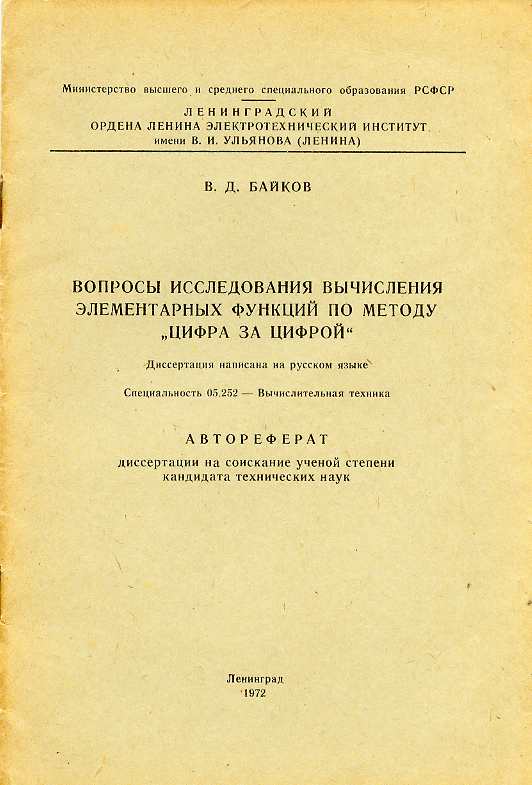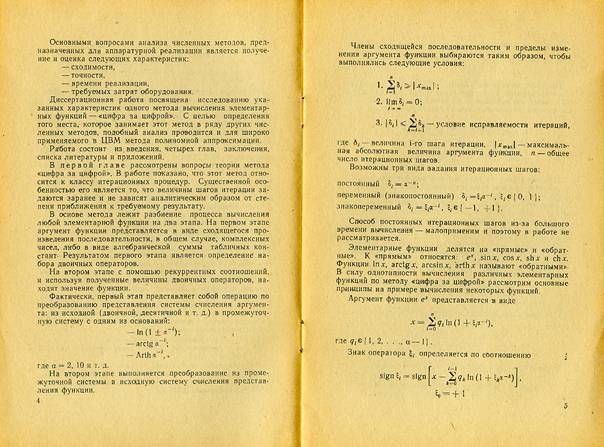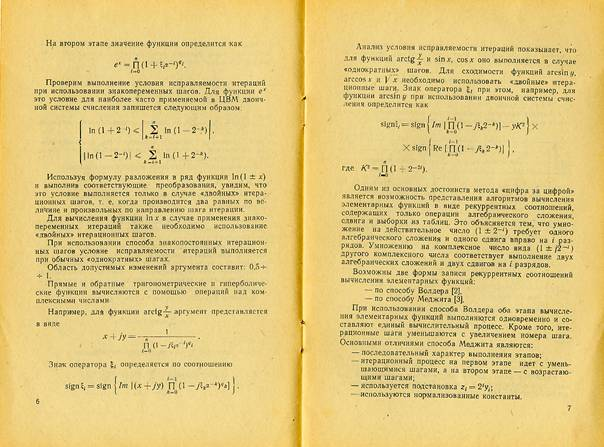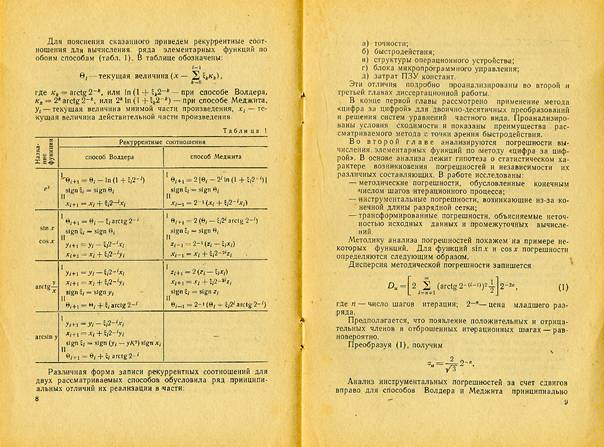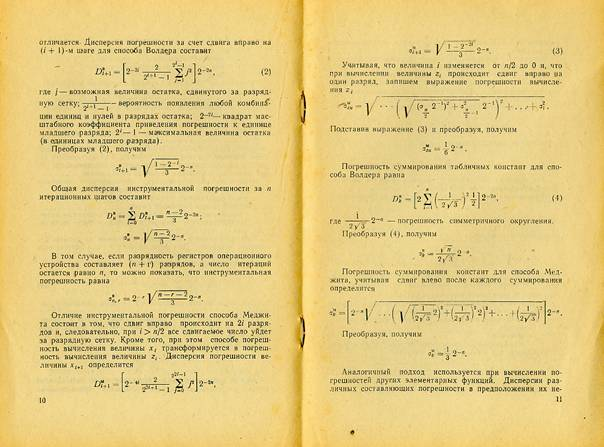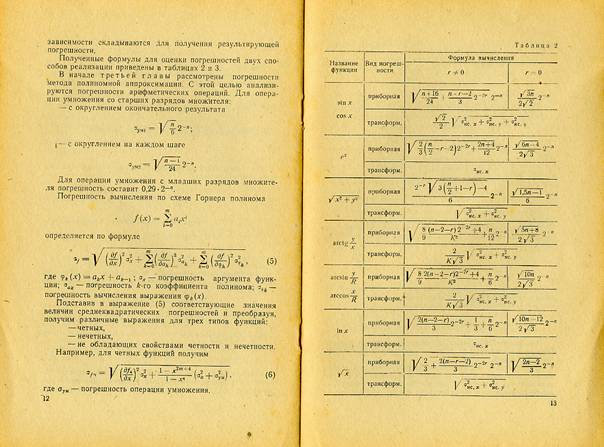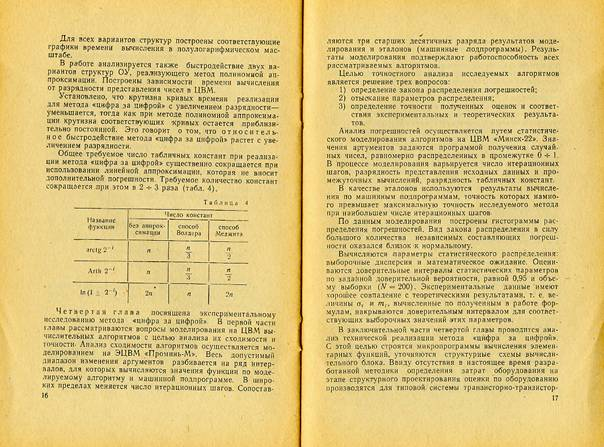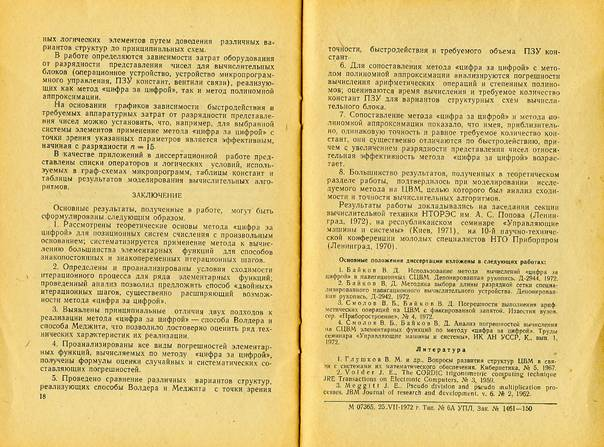All these results in more details were represented in my PhD thesis, in 1972
(150 pages)  and later, more expanded in the book (coauthor: Vladimir
Smolov) : "Hardware implementation of the elementary functions in computers"
(in Russian: Apparaturnaja realizatsija elementarnikh funktsij v CVM),
published in 1975 by
Leningrad State University in 10 000 copies.